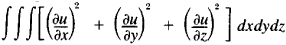# Dirichlet Integral

Also found in: Wikipedia.
The following article is from The Great Soviet Encyclopedia (1979). It might be outdated or ideologically biased.

## Dirichlet Integral

(named for P. G. L. Dirichlet), the name of several types of integrals.

(1) The integral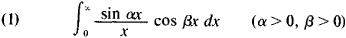This Dirichlet integral is also called the Dirichlet discontinous factor and is equal to π/2 for β < a, to β/4 for β = α, and to 0 for β > α. Thus, Dirichlet integral (1) is a discontinous function of parameters α and β. Dirichlet used the integral (1) in his studies of the attraction of ellipsoids. However, this integral appeared earlier in the works of J. Fourier, S. Poisson, and A. M. Legendre.

(2) The integral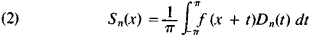where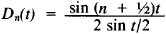is the so-called Dirichlet kernel. This Dirichlet integral is equal to the nth partial sum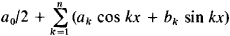of a Fourier series of the function f(x). Formula (2) is one of the most important formulas in the theory of Fourier series; in particular, it enabled Dirichlet to show that the Fourier series of a function with a finite number of maxima and minima converges at every point.

(3) The integral